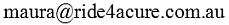Or you can send an email to0427 671 229

Or contact Esther at

esther@ride4acure.com.au

0400378427

Thanks!

```

Name:

Email:

Subject:

//<![CDATA[
function checkForm( n )
{
var f = new Array();
f = document.getElementById('tcf_sender' + n).value;
f = document.getElementById('tcf_email' + n).value;
f = document.getElementById('tcf_subject' + n).value;
f = document.getElementById('tcf_msg' + n).value;
f = f = f = f = f = '-';

var msg = "";
for ( i=0; i < f.length; i++ )
{
if ( f[i] == "" )
}
if ( !isEmail(f) )
msg += "Wrong Email.\nWrong Email.";
if ( msg != "" )
{
return false;
}
}
function isEmail(email)
{
var rx = /^([^\s@,:"<>]+)@([^\s@,:"<>]+\.[^\s@,:"<>.\d]{2,}|(\d{1,3}\.){3}\d{1,3})\$/;
var part = email.match(rx);
if ( part )
return true;
else
return false
}
//]]&gt;

```